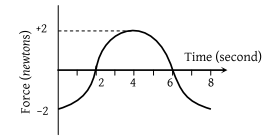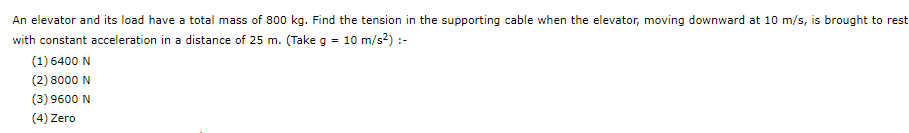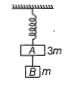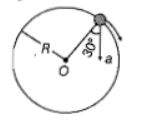A ball of mass m falls vertically to the ground from a height h1 and rebound to a height h2. The change in momentum of the ball on striking the ground is

(1) $mg\left({h}_{1}-{h}_{2}\right)$

(2) $m\left(\sqrt{2g{h}_{1}}+\sqrt{2g{h}_{2}}\right)$

(3) $m\sqrt{2g\left({h}_{1}+{h}_{2}\right)}$

(4) $m\sqrt{2g}\left({h}_{1}+{h}_{2}\right)$

Concept Questions :-

Application of laws
High Yielding Test Series + Question Bank - NEET 2020

Difficulty Level:

A force-time graph for a linear motion is shown in figure where the segments are circular. The linear momentum gained between zero and 8 second is1. $-2\pi \text{\hspace{0.17em}}newton×second$

2. $Zero\text{\hspace{0.17em}}newton×second$

3. $+4\pi \text{\hspace{0.17em}}newton×second$

4. $-6\pi \text{\hspace{0.17em}}newton×second$

Concept Questions :-

Newton laws
High Yielding Test Series + Question Bank - NEET 2020

Difficulty Level:Concept Questions :-

Application of laws

Difficulty Level:

Two blocks A and B of masses 3m and m respectively are connected by a massless and inextenisible string. The whole system is suspended by a massless spring as shown in figure. The magnitudes of acceleration of A and B  immediately after the string is cut, are respectively1. $g,\frac{g}{3}$

2. $\frac{g}{3},g$

3. $g,g$

4. $\frac{g}{3},\frac{g}{3}$

Concept Questions :-

Spring force
High Yielding Test Series + Question Bank - NEET 2020

Difficulty Level:

One end of the string of length l is connected to a particle of mass m and the other end is connected to a small peg on a smooth horizontal table. If the particle moves in circle with speed v, the net force on the particle (directed towards centre) will be (T represents the tension in the string)

1. T

2. $T+\frac{m{v}^{2}}{l}$

3. T-$\frac{m{v}^{2}}{l}$

4. Zero

Concept Questions :-

Uniform circular motion
High Yielding Test Series + Question Bank - NEET 2020

Difficulty Level:

A spring of force constant k is cut into lengths of ratio 1:2:3. They are connected in series and the new force constant is ${k}^{\text{'}}$. If they are connected in parallel and force constant is  is

(a) 1:6

(b) 1:9

(c) 1:11

(d) 6:11

Concept Questions :-

Spring force
High Yielding Test Series + Question Bank - NEET 2020

Difficulty Level:

In the given figure, represents the total acceleration of a particle moving in the clock wise direction in a circle of radius R=2.5 m at a given instant of time. The speed of the particle is(a) 4.5 m/s

(b) 5.0 m/s

(c) 5.7 m/s

(d) 6.2 m/s

Concept Questions :-

Uniform circular motion
High Yielding Test Series + Question Bank - NEET 2020

Difficulty Level:

A car is negotiating a curved road of radius R. The road is banked at angle $\mathrm{\theta }$. The coefficient of friction between the tyres of the car and the road is ${\mathrm{\mu }}_{\mathrm{s}}$. The maximum safe velocity on this road is

1. $\sqrt{\mathrm{gR}\left(\frac{{\mathrm{\mu }}_{\mathrm{s}}+\mathrm{tan\theta }}{1-{\mathrm{\mu }}_{\mathrm{s}}\mathrm{tan\theta }}\right)}$

2. $\sqrt{\frac{\mathrm{g}}{\mathrm{R}}\left(\frac{{\mathrm{\mu }}_{\mathrm{s}}+\mathrm{tan\theta }}{1-{\mathrm{\mu }}_{\mathrm{s}}\mathrm{tan\theta }}\right)}$

3. $\sqrt{\frac{\mathrm{g}}{{\mathrm{R}}^{2}}\left(\frac{{\mathrm{\mu }}_{\mathrm{s}}+\mathrm{tan\theta }}{1-{\mathrm{\mu }}_{\mathrm{s}}\mathrm{tan\theta }}\right)}$

4. $\sqrt{{\mathrm{gR}}^{2}\left(\frac{{\mathrm{\mu }}_{\mathrm{s}}+\mathrm{tan\theta }}{1-{\mathrm{\mu }}_{\mathrm{s}}\mathrm{tan\theta }}\right)}$

Concept Questions :-

High Yielding Test Series + Question Bank - NEET 2020

Difficulty Level:

What is the minimum velocity with which a body of mass m must enter a vertical loop of radius R so that it can complete the loop?

(a)$\sqrt{2\mathrm{gR}}$
(b)$\sqrt{3gR}$
(c)$\sqrt{5gR}$
(d)$\sqrt{gR}$

Concept Questions :-

Non-Uniform vertical circular motion
High Yielding Test Series + Question Bank - NEET 2020

Difficulty Level:

A particle of mass 10g moves along a circle of radius 6.4 cm with a constant tangential acceleration. What is the magnitude of this acceleration, if the kinetic energy of the particle becomes equal to 8x10-4 J by the end of the second revolution after the beginning of the motion?

(a) 0.15 m/s2
(b) 0.18 m/s2
(c) 0.2 m/s2
(d) 0.1 m/s2

Concept Questions :-

Uniform circular motion